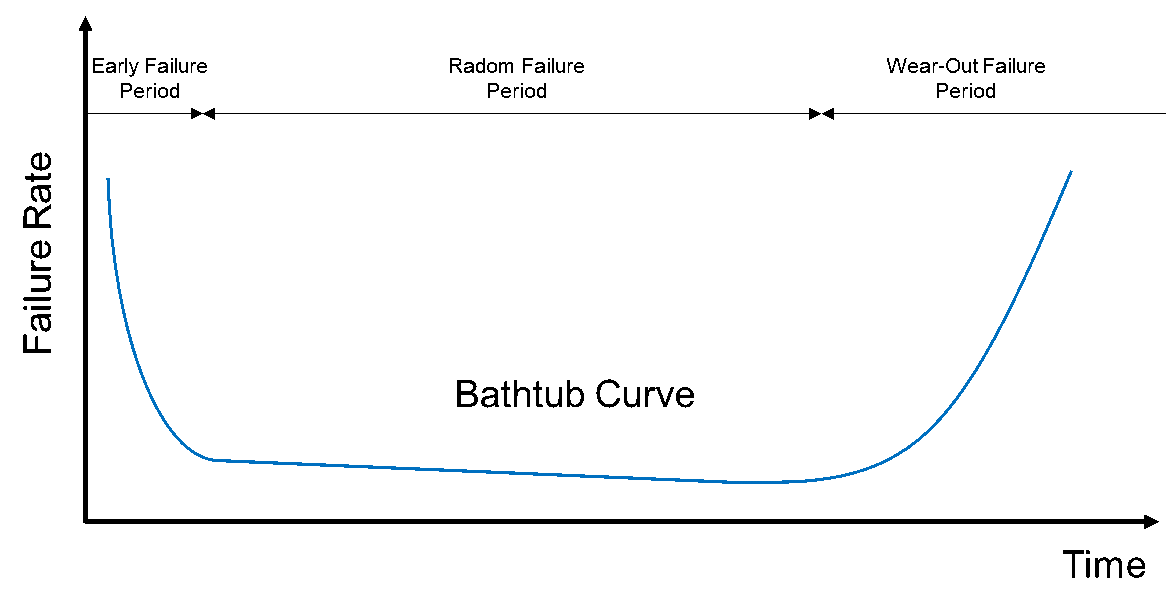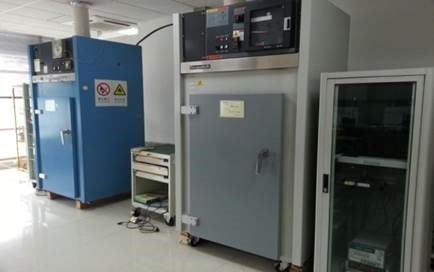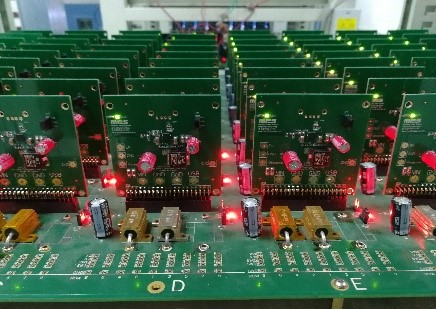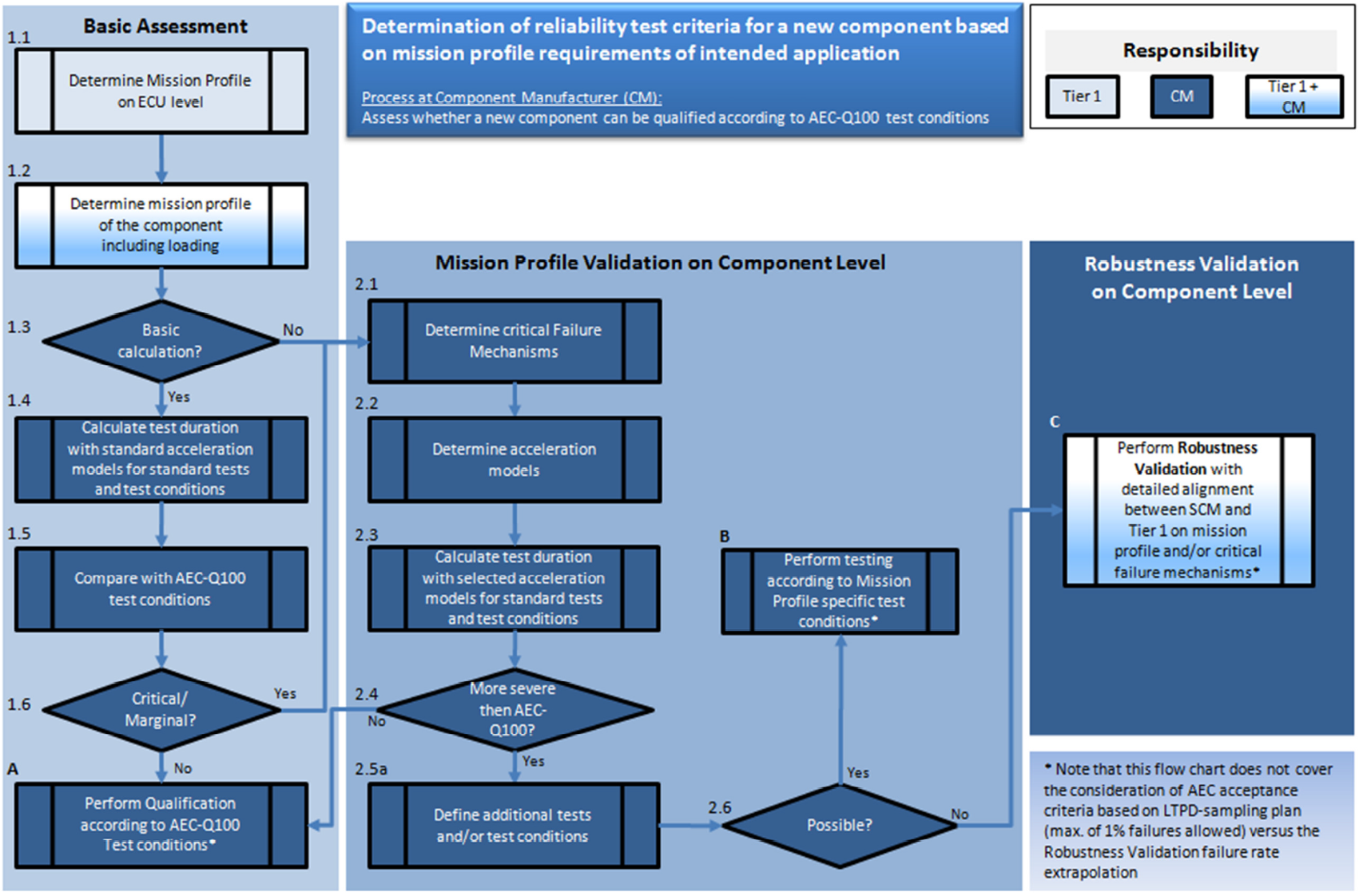# 汽车电子产品的可靠性测试始终围绕任务配置文件进行## 简介

• 电子元器件在其使用寿命期间可能会面对哪些类型的应力？
• 谁负责评定 IC 的可靠性能力？
• 如何应用可靠性“加速模型”来评估IC可靠性测试满足寿命评估需求?## 了解加速因子

HTOL测试由JEDEC标准JESD22-A108 定义。一组231个单元需要在125°C下运行1,000小时。该测试使用 Arrhenius 模型来确定温度加速因子(Af)，它提供所需的测试时间 (tt) 以模拟实际使用的等效时间。表1给出了一个任务配置文件的范例，该范例用来模拟平均结温87℃条件下，运行12000小时。Tj是硅器件的结温，对于有较大功耗的功率IC而言，结温需要被特别考虑，因为环境温度Ta往往远低于结温Tj。

 加载 操作 热机械 任务配置文件输入 tU = 12,000hr（15年的平均使用时间） $$A_f = exp \left[\frac{E_a}{K_B} \times \left(\frac{1}{T_u}-\frac{1}{T_t}\right)\right]$$ TU = 87°C （使用环境中的结温） nU = 54,750cls （使用超过 15 年的发动机开/关循环次数） ∆TU = 76°C （使用环境中的平均热循环温度变化） 应力测试 高温工作寿命(HTOL) 温度循环(TC) 应力条件 Tt = 125°C (测试环境中的结温) ∆Tt = 205°C （测试环境中的热循环温度变化：-55°C至+150°C) 加速模型（所有温度单位为 K） 阿伦尼斯（Arrhenius） 也适用于高温存储寿命 (HSL)、NVM 耐久性、数据烘烤和运行寿命 (EDR) 科芬·曼森（Coffin Manson） $$A_f = \left(\frac{\Delta T_t}{\Delta T_u}\right)^m$$ 也适用于功率温度循环 (PTC) 模型参数 Ea = 0.7eV （活化能；0.7eV为典型值，实际值取决于失效机制，范围从 -0.2eV 到 1.4eV） kB = 8.61733 x 10-5eV/K (玻尔兹曼常数） m = 4 （Coffin Manson 指数；4 表示硬质合金中的裂纹，实际值取决于失效机制，范围从表示韧性材料的1到表示脆性材料的9） 计算的测试时间 tt = 1,393hr(测试时间) $$t_t = \frac{t_u}{A_f}$$ nt = 1,034cls(测试循环次数) $$n_t=\frac{n_u}{A_f}$$ Q100测试时间 1,000hr 1,000cls

## 任务配置文件计算

 配置文件 主动 被动 TJ (°C) 时间(h) TJ (°C) 时间(h) -40 45 -40 346 -20 45 15 21168 40 855 25 42336 50 3150 35 21168 60 4950 40 1382 70 9000 86400 80 11250 90 6750 100 4950 110 2700 120 1170 125 135 45000 合计 131400 (15年寿命)

$$Af=exp⁡\Biggl[\left(\frac{Ea}{kb}\right)\times\left(\frac{1}{273K-40K}-\frac{1}{273K+125K}\right)\Biggl]$$ $$Af=4184927.76$$

$$Af=\frac{tu}{tt}$$ $$tt=\frac{tu}{Af}$$ $$tt=\frac{45h}{4184927.76}$$ $$tt=0.000107h$$## 任务配置文件定义